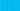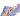PricingResources

# Understanding the gross margin formula

Written by

How do you measure a company's efficiency? One indicator is the gross profit margin, which shows how much profit is left over after a company has paid off all direct expenses. Keep reading to learn more about the gross profit margin formula and how it's used in business.

## What is the gross profit margin?

The gross profit margin is an important figure to track a company's sales figures and efficiency. It subtracts the cost of goods sold (COGS) from the business's total revenue to show what remains. This can then be converted into a percentage using the gross margin formula, which is easier to track over time.

There are many ways to track profit, so it's important to distinguish between gross profit margin and other types of margins such as:

• Net profit margin

• Operating profit margin

• Pre-tax profit margin

Each of these weighs different cost factors into the equation. For example, a net profit margin considers all expenses involved in product production, while the operating profit margin is calculated without tax.

Calculating gross margin allows a business to track its profitability over time. It doesn't include administrative costs like an operating profit margin, which means that it highlights profit purely after subtracting the cost of goods sold.

## How to calculate the gross profit margin formula

Here's how to calculate the gross margin index formula:

The result of the gross profit margin formula is typically represented as a percentage. To arrive at this percentage, use the following formula:

By contrast, gross profit is expressed in pounds and involves the following calculation:

You can plug some numbers in to see how this looks. Imagine a start-up candle business has generated \$100,000 in revenue during the financial year. The cost of goods sold was \$60,000 during this same period, including the cost of all labour, raw materials, packaging costs, and overheads.

The business's gross margin index formula is as follows:

Use the gross margin calculation formula to express this in a percentage:

The gross profit margin for the financial year is 40%. Calculate its gross profit in GBP like so:

## How to interpret the gross margin analysis formula

The gross margin calculation formula is only one piece of the puzzle. The business above may be wondering if the 40% gross profit margin is good enough or if they could be doing more to increase profit. To analyse gross margins, there are a few considerations to bear in mind.

First, it's essential to realise that a business's gross profit margin should only be compared with other companies within the same industry. Service-oriented companies usually have the highest profit margins because they don't require manufacturing costs. As a result, you can't accurately compare a product-oriented business with a service-oriented business. If the candle industry's average profit margin is 30%, the company we looked at above would be doing very well by hitting margins of 40%. However, if the average is more like 60%, it needs to find a way to become more competitive.

The second factor to consider when looking at the gross margin analysis formula is the company's age. Gross profit margins tend to be lower for start-ups because there are more costs involved, and it takes time to build a reliable customer base.

Finally, keep in mind that a company's gross margins should remain relatively stable over time. If there are significant fluctuations, this could indicate accounting irregularities or mismanagement, so any sudden jumps should warrant an investigation.

## We can help

GoCardless helps you automate payment collection, cutting down on the amount of admin your team needs to deal with when chasing invoices. Find out how GoCardless can help you with ad hoc payments or recurring payments.## Interested in automating the way you get paid? GoCardless can help

Contact sales

Sales

Contact Sales

+1(415) 523-2279

Support

help@gocardless.com

+1 (628) 241-0044